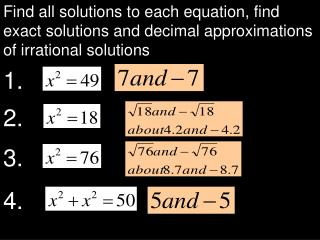Download Presentation2 .

Loading in 2 Seconds...

# 2 . - PowerPoint PPT Presentation

Find all solutions to each equation, find exact solutions and decimal approximations of irrational solutions. 1. 2. 3. 4. 1. 2. 3. 4. 1. 2. 3. 4. 0.176(2.4). 4224. 0.4224. 1. 2. 3. 4. 1. 2. 3. 4. or about 3.9. 64. 8. 1 5. 7. 4 9.I am the owner, or an agent authorized to act on behalf of the owner, of the copyrighted work described.
Download Presentation## 2 .

An Image/Link below is provided (as is) to download presentation

Download Policy: Content on the Website is provided to you AS IS for your information and personal use and may not be sold / licensed / shared on other websites without getting consent from its author.While downloading, if for some reason you are not able to download a presentation, the publisher may have deleted the file from their server.

- - - - - - - - - - - - - - - - - - - - - - - - - - E N D - - - - - - - - - - - - - - - - - - - - - - - - - -
Presentation Transcript
1. Find all solutions to each equation, find exact solutions and decimal approximations of irrational solutions 1. 2. 3. 4.

2. 1. 2. 3. 4.

3. 1. 2. 3. 4.

4. 0.176(2.4) 4224 0.4224

5. 1. 2. 3. 4.

6. 1. 2. 3. 4.

7. or about 3.9 64 8 15 7 49

8. Find the exact length of the missing side of each right triangle. Also find a decimal estimate of any irrational length. 3. 2. 7 1. 8 2 3 7 8 4. 5 9

9. Find the exact length of the missing side of each right triangle. Also find a decimal estimate of any irrational length. 3. 2. 7 1. 8 2 3 7 8 4. 5 9

10. Pattern: Examples:

11. Create two equivalent expression: Without exponents A single exponential expression Example 1:

12. Create two equivalent expression: Without exponents A single exponential expression Example 1: Example 2:

13. Create two equivalent expression: Evaluate Write as a single number with an exponent 1. 2. 4. 3.

14. ? ?

15. Evaluate: 1. 2. 3.

16. or about 6.2 64 8 25 5 39

17. Find the exact length of the missing side of each right triangle. Also find a decimal estimate of any irrational length. 3. 2. 8 1. 8 5 2 8 8 4. 5 10

18. Find the exact length of the missing side of each right triangle. Also find a decimal estimate of any irrational length. 3. 2. 8 1. 8 5 2 8 8 4. 5 10